## FLOTRAN Basics

FLOTRAN is a finite element analysis program for solving fluid flow and conjugate heat transfer problems.

The governing equations solved by FLOTRAN are the Navier-Stokes equations combined with the continuity equation, the thermal transport equation, and constitutive property relationships.
This equation represents a number of fluid flow equations: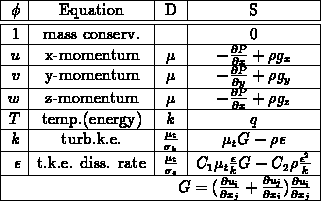###
Turbulent Model

As the Reynolds number increases, the flow becomes unstable and start to form eddies. This flow phenomenon is characterized as turbulent flow. The forming and disappearing of eddies change instantaneously and randomly in small scale. It would require very small time steps and very fine meshes to model the full time-dependent, small scale vortices of the Navier-Stokes equations. FLOTRAN uses themodel to approximate the turbulent flow.

Themodel uses time-averaging to obtain fluid flow equations. The velocity is expressed in terms of a mean velocity and a fluctuating velocity.Integrate over a small time scale:
Rewrite the compressible Navier-Stokes euqations in tensor form:
Perform time-averaging integration on above equation over the small time scale:
The time-averaged Navier-Stokes equations becomes:
are the time-averaged mean velocities. Additional advection terms are Reynolds stresses:
Introduce turbulent viscosity
and define the effective viscosity
:
Turbulent Navier-Stokes euqations in tensor form:
### Spalding and LaunderModel

Turbulent kinetic energy
Turbulent kinetic energy dissipation rate
Length scale related to turbulent kinetic energy
Turbulent viscosity
The source terms include the pressure gradient and the buoyancy forces and can also include additional source terms such as distributed resistances (head loss factors, friction factors, or permeability).

The density may be taken to be a function of temperature and/or pressure.. Several different polynomial relationships are available. Coefficients are calculated based on a user-selected set of data points.

For cases at high Reynolds number a two-equation turbulence model is used. In the turbulent case the effects of the time-varying velocity fluctuations are accounted for by an eddy viscosity model. Turbulent viscosity and turbulent thermal conductivity components are calculated based on the turbulent kinetic energy and turbulent kinetic energy dissipation rate and added to the corresponding laminar properties. The solution of partial differential equations based on the conservation of momentum provide the values ofand. The result is a spatially varying effective viscosity and effective conductivity field.

The governing equations are solved in sequential fashion using a segregated velocity-pressure solution algorithm. The process features the development of a Poisson-like pressure equation based on conservation of mass. An approximate solution to the momentum equations provides the forcing function for the pressure equation and the resulting pressure field is used to update velocity to ensure conservation of mass. This method features equal order elements for pressure and velocity and so all dependent variables are obtained for the same set of finite element nodes.

A monotone streamline upwind method is used to handle the nonlinear advection terms in the governing transport equations. The velocity field existing at any point in the solution process is used to identify which node(s) in a given element are downwind nodes. Streamlines from this velocity field are traced from the downwind nodes upstream to see where they entered the element. The advection term for that element is then calculated using conditions at that location. This method provides a stable solution while minimizing numerical diffusion, an important consideration in any approach to a mixing problem.

## FLOTRAN Features

• Steady-State or Transient Solution Algorithm
• Equal Order Approximation for Velocity and Pressure
1. No staggered grids
2. No mixed order interpolation
• Monotone Streamline Upwind Appproximation for Advection Terms
1. Increased accuracy over conventional upwind
2. Stable at high Reynolds numbers
• Minimal Execution Time Requirements
1. Segregated sequential solution
2. Iterative solution methods
• Minimal Storage Requirements
1. Bandwidth independent
2. In-core solution method

## FLOTRAN Capabilities

• 2-D or 3-D Geometries
• Axisymmetric Geometries
• Laminar or Turbulent Flow and/or Heat Transfer
• Forced, Free, or Mixed Convection
• Conduction
• Conjugate Heat Transfer
• Distributed Flow Resistances
• Porous Media
• Incompressible or Compressible Flow
• Total Energy Equation
• Global Convergence Status

• Boundary Conditions:
1. Prescribed Nodal Values - Velocity, Pressure, Temperature,Turbulent Kinetic Energy, Turbulent Energy Dissipation.
2. Prescribed Heat Flux
3. Prescribed Heat Source
4. Prescribed Film Coefficient
6. Symmetry
7. Periodic Boundaries

## Getting Started Using FLOTRAN

FLOTRAN has been integrated with ANSYS. This integration allows users to build the finite element models using ANSYS PREP7 and to pass the model data to flotran for the various analyses. Once the FLOTRAN analyses are done, the data can then be transferred to the ANSYS postprocessor, POST1.

IDEAS and PATRAN can also be used to pre- and postprocess finite element models for FLOTRAN analyses.

In this course, we will use only ANSYS for pre- and postprocesses.

### FLOTRAN Processes

• Preprocessing (or model generation and B.C./I.C.) - ANSYS,
• Solution - FLOTRAN,
• Postprocessing (or results evaluation) - ANSYS.

## FLOTRAN Modeling

Only two element types are valid for FLOTRAN analyses. FLOTRAN uses the MAT element attribute to distinguish fluid elements from solid elements. Fluid elements must have MAT = 1, and solid elements elements are identified by MAT > 1. The REAL ATTRIBUTE is to flag elements with distributed resistance.

### Modeling Constraints

• Element Types
1. Quad and triangles (2D) - PLANE55
2. Hex and tet (3D) - SOLID70
• Element Material Number
1. MAT = 1 - Fluid Elements
2. MAT > 1 - Solid Elements
• Cartesian Coordinates
• Rotating Coordinates
• Cylindrical Coordinates

### Modeling Guidelines

• Examine the solution domain for possible symmetries
• Sketch the geometry for planning the geometry model and the finite element model
• Anticipate the analysis requirements
1. Regions of high gradients in the solution variables. These regions usually require finer meshes.
2. Important Boundaries such as flow inlets, flow exits, heated areas, and constant temperature boundaries.
3. Mesh size requirements to be considered with maximum limit of nodes and CPU and storage requirements.
• Sketch the proposed mesh
• Construct the geometry and elments with top-down or bottom-up technique

### Boundary Condition Types

• Symmetry boundaries
• Geometry half symmetry or Mirror image
• Align with a coordinate axis
• Zero normal velocity on the bounaries
• Inflow boundaries - specify either inlet velocity or pressure
• Outflow boubdaries - specify pressure only
• Wall boundaries - zero flow velocity on the wall
• Periodic Boundaries - corresponding boundaries with identical flows
• Thermal boundary conditions - do not affect flow boundary conditions
• Initial conditions - specified with separate boundary condition in each LOAD STEP(ANSYS)

### Optimum Order for Setting Boundary conditions

• Symmetry boundaries
• Inflow boundaries
• Outflow boundaries
• Wall boundaries

When a boundary condition is repeatedly specified, the latter overwrite the former one. Unspecified flow boundaries are treated as natural boundary conditions where mass can enter or leave the boundaries.

## FLOTRAN Modeling - Example 1

```   /TITLE,Flow through a Curved Channel
/UNITS,SI  ! SI units
/PREP7     ! Begin PREP7 preprocessing
ET,1,55    ! Plane55 Element type
! Define pipe dimensions
D=20   ! channel width
R=0.5*D
RI=40  ! Radius of curved center line of channel
D4=D*4 ! Four times half channel width
RR=R*R
V0=200
K,1,,-R
K,2,,R
K,3
L,1,2
/pnum,line,1
/pnum,kp,1
/pnum,area,1
/pnum,node,1
lplot
LESIZE,1,,,16,-4
/triad,off  ! turn off coordinate traid at origin
KPLOT
K,4,D4+RI
K,5,D4+RI,D4*2+RI
L,3,4
L,4,5
LFILL,2,3,RI
LESIZE,2,,,32,0.5
LESIZE,3,,,48,2.5
LESIZE,4,,,32
LPLOT
APLOT
AMESH,ALL
/pnum,node,0
/pnum,kp,0
/pnum,elem,0
EPLOT
DOF,VX,VY,VZ,PRES,ENKE,ENDS
FLDA,1S,JB,cflow
FLDA,1S,IT,100
FLDA,1S,RS,T
FLDA,1S,TB,T
NSEL,s,loc,x
NPLOT
/pbc,all,,a
D,all,vy
GET,NMAX,NODE,,NUM,MAX
DO,I,1,NMAX
RSQ=NX(I)**2+NY(I)**2
VZ0=V0*(1.0-(RSQ/RR)**2)
D,I,VX,VZ0
ENDDO
nsel,s,loc,y,RI+D4*2
d,all,pres
LSEL,S,LINE,,6,7
LSEL,A,LINE,,9,10
LSEL,A,LINE,,12,13
NSLL,S,1
D,ALL,VX,,,,,VY
lsel,all
NSEL,ALL
FLDA,2P,D0,9.98E-1
FLDA,2P,V0,0.01
FLDA,2P,C0,6.04E-3
FLDA,2P,CP,4.199
FLDA,2S,NP,1.01325E6
FLWRITE,ALL
FINISH
/EXIT
```

## FLOTRAN Modeling - Example 2

```   /TITLE,Flow through a Curved Pipe
/UNITS,SI
/PREP7
ET,1,55
ET,2,70
! Define pipe dimensions
D=20    ! Pipe diameter
RI=40   ! Radius of curved center line of pipe
D4=D*4  ! Four times diameter length
RR=(0.5*D)**2
V0=200
PCIRC,0.4*D,,0,90
PCIRC,0.4*D,0.5*D,0,90
NUMMRG,ALL
/PNUM,line,1
/PNUM,area,1
/PNUM,kp,1
LPLOT
TYPE,1
LESIZE,1,,,8
LESIZE,2,,,8,2
LESIZE,3,,,8,0.5
LESIZE,5,,,4,2
LESIZE,7,,,4,2
APLOT
ESHAPE,2
AMESH,ALL
ARSYM,X,ALL
NUMMRG,ALL
ARSYM,Y,ALL
NUMMRG,ALL
NUMCMP,NODE
/pnum,node,1
TYPE,2
K,23,,,D4+RI
K,24,D4*2+RI,,D4+RI
L,3,23
L,23,24
lplot
LFILL,13,16,RI
LESIZE,13,,,16,0.25
LESIZE,18,,,32
LESIZE,16,,,24,5
/VIEW,1,1,1
VDRAG,ALL,,,,,,13,18,16
NUMMRG,NODE
ASEL,S,TYPE,,1
ACLEAR,ALL
/pnum,node,0
/pnum,kp,0
/pnum,elem,0
EPLOT
VSEL,S,TYPE,,2
NSLV,S,1
DOF,VX,VY,VZ,PRES,ENKE,ENDS
NSEL,S,LOC,Z
NPLOT
/pbc,all,,a
D,ALL,VX  ! Flow at inlet
D,ALL,VY
GET,NMAX,NODE,,NUM,MAX
DO,I,1,NMAX
RSQ=NX(I)**2+NY(I)**2
VZ0=V0*(1.0-(RSQ/RR)**2)
D,I,VZ,VZ0
ENDDO
NSEL,S,LOC,X,RI+D4*2
NPLOT
D,ALL,PRESS  ! Pressure at outlat
ASEL,S,EXT  ! Select external walls
ASEL,U,AREA,,1,8  ! Exclude inlet
ASEL,U,LOC,X,RI+D4*2  ! Exclude outlet
NSLA,S,0  ! Select all nodes on the walls
D,ALL,VX,,,,Vy,VZ  ! No slip on walls
ASEL,ALL
NSEL,ALL
ESEL,S,type,,2
FLDA,1S,JB,cpipe
FLDA,1S,IT,50
FLDA,1S,RS,T
FLDA,1S,TB,T
FLDA,2S,NP,1.01325E6
FLDA,2P,D0,9.98E-1
FLDA,2P,V0,0.01
FLDA,2P,C0,6.04E-3
FLDA,2P,CP,4.199
FLWRITE,ALL
SAVE
```

## FLOTRAN Setup

ANSYS incorporates FLOTRAN menus to setup FLOTRAN analysis control parameters within PREP7. The control data, the model geometry, the boundary conditions, and initial conditions are then written into separate FLOTRAN files for FLOTRAN to use.

FLOTRAN files for a particular problem use a common file name (jobname) with different extensions to identify the types of file. The jobname must be consistent with the file naming convention and is limited to 8 characters. If a jobname consists of digits as the trailing charaters, the digits are treat as a case identifier and will be stripped from the jobname.

```  jobname.xgm    transfer geometry file written by ANSYS PREP7
jobname&.xbc   transfer B.C. file written by ANSYS PREP7
jobname.geom   FLOTRAN translated geometry file
jobname&.bc    FLOTRAN boundary condition file
jobname&.run   FLOTRAN run control file
jobname&.res   FLOTRAN results file
jobname&.rso   FLOTRAN backup results file
jobname&.rsw   FLOTRAN wall file
jobname&.prt   FLOTRAN print file

Transient Runs
jobname&.xic   transfer initial condition file
jobname&.ic    FLOTRAN initial condition file
jobname&.#     FLOTRAN intermediate transient results file #
```

## FLOTRAN Screens

```   <1S>   Basic Run Data
<2S>   Properties
<2P>   User Fluid Data
<3S>   Turbulence
<4S>   Relaxation Parameters
<5S>   Solution Control
<6S>   Transient Control
<7S>   Rotational Terms

Quit, No Changes
Write File and Exit

ENTER CODE AND VALUE (e.g.  IT 100)
```

### Screen 1S - Basic Run Data

```   Create Post File          F   Flow Termination         0.0E+00
Iterations                50  Energy Termination      0.0E+00
Output Frequency          5   Print U Velocity         F
Restart                   F   Print V Velocity         F
Batch Output              F   Print WVelocity          F
Flow Analysis             T   Print Pressure           F
Turbulent                 T   Print Temperature        F
Compressible Solution     F   Print Turb Kin Energy    F
Thermal Analysis          F   Swirling Flow            F
Print Turb Dissipation    F   Output Residuals         F
```

### Screen 2S - Properties

```   Fluid                    CONSTANT
Reference Pressure       1.0133E+6
Nominal Temperature      2.9300E+2
Ambient Temperature      2.9300E+2
Stagnation Temperature   2.9300E+2
Print Density            F
Print Viscosity          F
Print Conductivity       F
X Acceleration            0.000E+00
Y Acceleration           0.000E+00
Z Acceleration           0.000E+00
Variable Density         F
Variable Viscosity       F
Variable Conductivity    F
Property Update Freq.    1
```

### Screen 2P - User Fluid Data

```           (Fluid CONSTANT, LIQUID or GAS only)

Base
Value              Coeff
Density          9.9800E-1      0.0000E+0
0.0000E+0
0.0000E+0
Viscosity        1.0000E-2      0.0000E+0
0.0000E+0
0.0000E+0
Conductivity     6.0400E-3      0.0000E+0
0.0000E+0
0.0000E+0
Specific Heat    4.1990E+0
Ratio CP/CV      1.4000E+0
```

### Variable Properties

Functional Form for Gas

Density: Ideal Gas Equation (
)
Viscosity: Sutherland's Formula
Conductivity: Sutherland's Formula
Functional Form for Liquid
Viscosity: Exponential
Conductivity: Exponential### Screen 3S - Turbulence Parameters

```  Inlet Intensity      0.0100     Print Eff Viscosity       F
Inlet Scale Factor   0.0100     Print Eff Conductivity    F
Turbulence Ratio     1.0E+03
Schmidt Number
Temperature          1.0000     Turb Kinetic Energy       1.0000
Turb Dissipation Rate     1.3000

Turbulence Parameters
CMu    0.0900     Kappa    0.4000     E    9.000
C1     1.4400     C2       1.9200     A    26.00
```

### Screen 4S - Relaxation Parameters

```  U Velocity Relaxation       0.500
V Velocity Relaxation       0.500
W Velocity Relaxation       0.500
Pressure Relaxation         0.500
Turb Kin Energy Relax       0.500
Turb Dissipation Relax      0.500
Temperature Relaxation      0.500
Density Relaxation          0.500
Viscosity Relaxation        0.500
Conductivity Relaxation     0.500
Eff Viscosity Relax         0.500
Eff Conductivity Relax      0.500

Momentum Inertia            1.E+15
Pressure Inertia            1.E+15
Turbulence Inertia          1.E+15

Artificial Viscosity        0.E+00
```

### Screen 5S - Solution Control

```	                        Pressure       Energy
Semi-direct Solution      T          F
Convergence Criteria      1.E-07     1.E-05
Maximum Iterations        300        300

TDMA Iterations
Momentum              1      Kinetic Energy          10
Pressure              100    Dissipation Rate        10
Temperature           25
[VM=1,P=2,K=3,E=4,T=5,  Rho=6]
Debug Print Level     0      Solution Error Est.      0
```

### Screen 6S - Transient Control

```  Transient Solution         F
Number of time steps       10
Time step                  -1.0000E+0
Stop Time                  1.0000E+0
Output Time Increment      1.000E+09
Output Step Increment      9999
```

### Screen 7S - Rotational Terms

```  X Omega (Rot. speed)      0.000E+00
Y Omega (Rot. speed)      0.000E+00
Z Omega (Rot. speed)      0.000E+00
X Rotat. Axis Offset      0.000E+00
Y Rotat. Axis Offset      0.000E+00
Z Rotat. Axis Offset      0.000E+00
```

## Running FLOTRAN

Once the finite element model has been prepared by ANSYS PREP7, (IDEASS or PATRAN,) you may submit it to FLOTRAN by entering:

flotran

and respond to the prompts.

FLOTRAN Production Dynamic

Version 2.1a Convex 6/01/93

This is the FLOTRAN (R) general purpose finite element computer program for fluid flow and heat transfer analysis. Neither Swanson Analysis Systems, Inc. nor any distributor supplying this program assume any responsibility for the validity, accuracy, or applicability of any results obtained from the FLOTRAN (R) program. Users must verify their own results.

1. Setup Run 2. Submit Run 3. Results Processing 4. Utilities 5. Exit
1

1. Geometry 2. Boundary Cond. 3. Initial Cond. 4. Run File 5. Exit
1

Enter FLOTRAN jobname (8 characters)
cflow

Translate geometry for cflow ? (Y or N)
y

Overwrite current geometry? (Y or N)
y

Enter preprocessor file name

Translate BC for cflow ? (Y or N)
y

Enter preprocessor file name

1. Setup Run 2. Submit Run 3. Results Processing 4. Utilities 5. Exit
5

Do not select "2. Submit Run" for running a batch job. Exit flotran and use the NIST modified FLORUN command to submit a FLOTRAN batch job:

florun.nist <jobname> batch [CPU hours] [Mem-size Mb]

## FLOTRAN Solution

A complete pass through all the equations is called a global iteration consisting of

1. Approximate solution of each momentum equation in sequential fasion.
2. Solution of the pressure equation.
3. Calculation of velocities to conserve mass.
4. Solution of the energy equation.
5. Update of the laminar properties.
6. Solution of the turbulence equations.
7. Update of the turbulent properties.

The progress of the solution is monitored by observing the rate of change of the solution from one global iteration to the next. The analyst can restart the analysis until satisfied that the rate of change of the solution is small enough.

The approximate solution of the momentum equation is obtained through the use of a tri-diagonal matrix algorithm. The momentum equations are relaxed to provide a stable solution.

The pressure equation is solved with a pre-conditioned conjugate gradient routine. An incomplete Choleski decomposition provides the preconditioning. The approach is particularly well suited for practical applications because the solver requires very little storage other than those associated with the non-zero matrix terms in the nonsymmetric momentum equation. The performance of the method is bandwidth independent and it is ideally suited towards use with irregular and unstructured finite element grids.

## Convergence Monitoring

```ITER   U-MOM       V-MOM       PRESS       ENERG       K           EPSILON
1   0.000E+00   0.000E+00   1.000E+00   0.000E+00   0.000E+00   0.000E+00
2   4.823E-02   4.731E-02   2.142E+00   0.000E+00   9.991E-01   6.062E-01
3   1.563E-02   1.633E-02   7.292E-01   0.000E+00   3.453E-01   3.430E-01
4   1.368E-02   1.582E-02   3.680E-01   0.000E+00   2.090E-01   2.421E-01
5   1.316E-02   1.520E-02   2.053E-01   0.000E+00   1.517E-01   1.907E-01
6   1.276E-02   1.487E-02   1.605E-01   0.000E+00   1.214E-01   1.587E-01
7   1.231E-02   1.463E-02   1.199E-01   0.000E+00   1.031E-01   1.370E-01
8   1.184E-02   1.421E-02   7.186E-02   0.000E+00   9.050E-02   1.215E-01
9   1.134E-02   1.423E-02   9.942E-02   0.000E+00   7.999E-02   1.073E-01
10   1.095E-02   1.385E-02   5.649E-02   0.000E+00   7.142E-02   9.527E-02

Global Convergence Statistics

U-MOM       V-MOM       PRESS       ENERG       K           EPSILON
Err   3.978E+03   4.579E+03   3.483E+06   0.000E+00   1.805E+08   1.130E+06
Sig   6.750E+03   8.080E+03   6.349E+06   0.000E+00   5.080E+08   1.476E+06

Total Mass Flow In    =   6356.2
Total Mass Flow Out   =   -6356.0

Total Energy Flow In  =   0.78201E+07
Total Energy Flow Out =   -0.78198E+07
```

## Results Evaluation

• Field Variable Information
1. Velocity
2. Pressure
3. Temperature
4. Total Temperature
5. Turbulent Kinetic Energy
6. Turbulent Dissipation Rate
7. Density
8. Molecular Viscosity
9. Effective Viscosity
10. Thermal Conductivity
• Calculated Field Quanities
1. Stream Function (2D)
2. Velocity Magnitude (3D)
3. Wall Film Coefficients
4. Wall Heat Fluxes
5. Pressure Coefficient
6. Mach Number
7. Total Pressure
8. Nodal Heat Fluxes
9. Nodal Film Coefficients
• Solution Reliability Statistics
1. Nodal Residuals
2. Nodal Error Indicators
• Graphic Output:
1. All of the imaging capabilities (e.g., contour plots, vector plots, etc.) All FLOTRAN variables can be used for postprocessing.
2. Massless Particle Tracking is available in the ANSYS and IDEAS programs.

## Post Processing Variables

```   VX     X direction Velocity
VY     Y direction Velocity
VZ     Z direction Velocity
PRES   Pressure
TEMP   Temperature
ENKE   Turbulent Kinetic Energy
ENDS   K. E. Dissipation Rate
NDEN   Nensity
NVIS   Viscosity
TCON   Fluid Thermal Conductivity
EVIS   Effective Viscosity
ECON   Effective Conductivity
TTOT   Total Temperature
STRM   Stream Function
PCOE   Pressure Coefficient
MACH   Mach Number
PTOT   Total Pressure
HFLX   Heat Flux
HFLM   Film Coefficient
```

## FLOTRAN Postprocessing

The FLOTRAN results file, <jobname&.res> is a unformatted binary file that can be read by the FLREAD command in ANSYS postprocessing.

Where ext is the extension of FLOTRAN filename.

```   res   Nodal results file for all degrees of freedom as well as properties
nqh   Heat flux and filem coefficients
eid   Error indicator file
```

### Plot Results:

```   PLNSOL, variable (Velocity, Temperature, Pressure, etc.)
```

### Plot velocity vectors:

```   PLVECT, V
```

### Plot graphs along a line path:

```   Define Path:
LPTATH,node1,node2,....node10
Define Name of Graph:
PDEF, User-define-name, Variable
Produce Graph:
PLPATH, User-define-name
```

### Integrate Pressures:

```   INTSRF, PRES
```

### Particles Tracing:

```   Define up to 50 points in problem domain
TRPOIN,x,y,z
TRPOIN,PICK
List existing points with TRPLIS
Delete points with TRPDEL

Execute particle tracing of any post processing variable
PLTRAC, FLUID, item, compon
```

### 3D Contour Plots:

```      /CTYPE,1
/EDGE,off
ASEL,S,EXT
NSLA,S,ALL,1
NSEL,INVE
PLNSOL,V,SUM
```

### 3D Cross Section Contour Plots

```      /TYPE,3,SECT
/FOCUS,3,RI,0,D4+RI
/VIEW,3,1,0,0
NSEL,s,loc,x,RI
ESLN,,0
PLNSOL,V,SUM
PLNSOL,PRESS
```
Transient results files use ANSYS POST26.

## FLOTRAN Postprocessing Example

```  RESUME,cpipe,db
/POST1
/WINDOW,1,FULL
/WINDOW,2,-.2,.4,-1,-.4
/WINDOW,3,.4,1,-1,-.4
/TLABEL,0.25,-.98,Z=D*4
/TLABEL,1,-.98,X=RI
/window,all,off
/CTYPE,1
nsel,s,loc,y,-99,0
esln,,0
/window,1,on
/VIEW,1,0.5,1,-0.5
PLNSOL,V,SUM
/noerase
/window,1,off
NSEL,ALL
/CTYPE
/window,2,on
/TYPE,2,SECT
/FOCUS,2,0,0,D4
/VIEW,2,0,0,1
nsel,s,loc,z,D4
esln,,0
PLNSOL,V,SUM
/window,2,off
/window,3,on
/TYPE,3,SECT
/FOCUS,3,RI,0,D4+RI
/VIEW,3,1,0,0
nsel,s,loc,x,RI
esln,,0
PLNSOL,V,SUM
```

## Error and Trouble Shooting

FLOTRAN error message indicates a fatal error. FLOTRAN performs a number of data checks to ensure that the problem posted is valid. There are, of course, many inputs that cannot be verified; users must verify them. Diverging solution, and unexpected results are often caused by invalid input.

### Common Causes of Divergence

• Incorrect problem setup
• Highly skewed or tapered finite elements
• Missing or duplicate nodes
• Incorrect or missing boundary conditions
• Unreferenced nodes
• Poorly defined problem domain
• Incorrect problem setup in FLOTRAN
• Inconsistent property information
• Invalid control parameters
• Tough fluids problem

### Trouble Shooting Guide

• Users may check the boundary conditions by setting both Flow and Thermal in Screen 1S to F(false) and Iteration to 0. The FLOTRAN will generate a jobname.prt that tabulates the mass flows at all inlets and outlets. If unexpected inlets/outlets are listed, eliminate them.
• Make sure information on Screen 2S and Screen 2P are realistic and consistent. For example, a temperature data given in
when it should be given in
may give an unrealistic density and cause divergence.
• If the divergence occurs on the first few iterations, it is usually either a modeling error or an incorrect FLOTRAN data specification. If a FLOTRAN analysis runs for a short time (5-10 iterations) where it appears to converge and then diverges, it may be caused by improper run parameters. For example, a laminar flow analysis on a high Reynold number situation may first converge then diverge.
• Reducing the relaxation parameters on Screen 4S may help the convergence. These parameters are set at 0.5 and may be reduced to 0.2-0.3 to better control the convergence. Another alternative is to lower the inertia number on Screen 4S.
• If these methods stiil fail to converge, it may be possible to pre-condition the problem. This is done by raising the viscosity an appropiate order of magnitude to obtain a laminar solution and then introducing the turbulence.

## FLOTRAN Applications

FLOTRAN has been applied to a variety of applications from a wide range of industries.

• AUTOMOTIVE

1. Study of air flow over vehicles
2. Passenger compartment flow
3. Fluid flow, conjugate heat transfer analysis of engine exhaust manifolds and water jackets - Flow through radiator passages
4. Flow analysis through various valve configurations

• AEROSPACE

1. External flow over various wing configurations
2. Internal flow analyses of nozzels and ducts
3. Fluid flow, heat transfer analysis of aircraft passenger compartments
4. Compressible flow analysis with shock/boundary layer interaction
5. Thermal analyses

• ELECTRONICS

1. Electronic cooling in a computer terminal
2. Conjugate heat transfer analysis of IC package
3. Heat transfer analysis of electronic fin array
4. Heat transfer analysis of a simulated card cage
5. Disk flow

• POWER

1. Thermally stratified turbulent pipe flow
2. Pressurized thermal shock
3. Natural convection cooling
4. Fluid bed reactor analysis
5. Valve analysis

• HVAC

1. Flow inside buildings
2. Flow in ducts
3. Flow in pipes
4. Flow through coolant passages
5. Heat exchanger analysis

• TURBO MACHINERY

1. Torque converter flow analysis
2. Flow through vaned and vaneless diffusers
3. Labyrinth seal flow